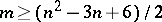# Graph circuit

(diff) ← Older revision | Latest revision (diff) | Newer revision → (diff)
An edge progression (closed edge progression) is called a Hamilton chain (Hamilton cycle) if it contains all the vertices of the graph and if it passes through each of them once. Several conditions sufficient for the existence of Hamilton cycles are known, such as: The graph has no loops or multiple edges and, for any two of its non-adjacent edges, the sum of their degrees is not less than the number of vertices in the graph; the graph is planar and quadruply-connected; the graph has no loops or multiple edges, and the numberof its vertices andof its edges satisfy the conditionsand. A graph is said to be Hamiltonian (Eulerian) if it has a Hamilton (Euler) cycle. A graph is said to be Hamilton-connected if any two of its vertices are connected by a Hamilton chain, and is said to be-Hamiltonian if any simple chain of lengthforms part of some Hamilton cycle. The well-known "travelling-salesman problemtravelling-salesman problem" is to find in a graph with edges labeled with non-negative numbers (lengths of edges) a Hamilton cycle with the smallest sum of edge lengths. This problem and other problems of graph circuits have various technological and economic applications.